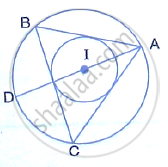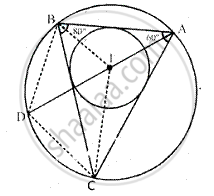Share

If I is the Incentre of Triangle Abc and Ai When Produced Meets the Circumcircle of Triangle Abc in Point D. If ∠Bac = 66° and ∠Abc = 80°. Calculate : - ICSE Class 10 - Mathematics

ConceptArc and Chord Properties - Angles in the Same Segment of a Circle Are Equal (Without Proof)

Question

If I is the incentre of triangle ABC and AI when produced meets the circumcircle of triangle ABC in point D. If ∠BAC = 66° and ∠ABC = 80°. Calculate :(i) ∠DBC  (ii) ∠IBC  (iii) BIC

SolutionJoin DB and DC, IB and IC
∠BAC  66°, ∠ABC  80°, I is the incentre of the ΔABC,
(i) since ∠DBC and ∠DACare in the same segment

∠DBC = ∠DAC
But, ∠DAC = 1 /2 ∠BAC = 1 /2 xx 66° = 33° =

∴ ∠DBC = 33°

(ii) Since I is the incentre of ∆ABC, IB bisects ∠ABC
∴ ∠IBC = 1/2 ∠ABC = 1 /2 xx 80° = 40°

(iii) ∠BAC = 66° and ∠ABC = 80°
In ∠ABC, ∠ACB = 180° - (∠ABC + ∠BAC)
⇒ ∠ACB = 180° - (80° + 66°)
⇒ ∠ACB = 180° - (156°)
⇒ ∠ACB = 34°
Since IC bisects the ∠C

∴∠ICB = 1/2 ∠C = 1/2xx  34° = 17°
Now in IBC
∠IBC + ∠ICB + ∠BIC =180°
⇒  40° +17° + ∠BIC = 180°
⇒  57° + ∠BIC = 180°
⇒ ∠BIC = 180° - 57°
⇒ ∠BIC = 123°

Is there an error in this question or solution?
Solution If I is the Incentre of Triangle Abc and Ai When Produced Meets the Circumcircle of Triangle Abc in Point D. If ∠Bac = 66° and ∠Abc = 80°. Calculate : Concept: Arc and Chord Properties - Angles in the Same Segment of a Circle Are Equal (Without Proof).
S